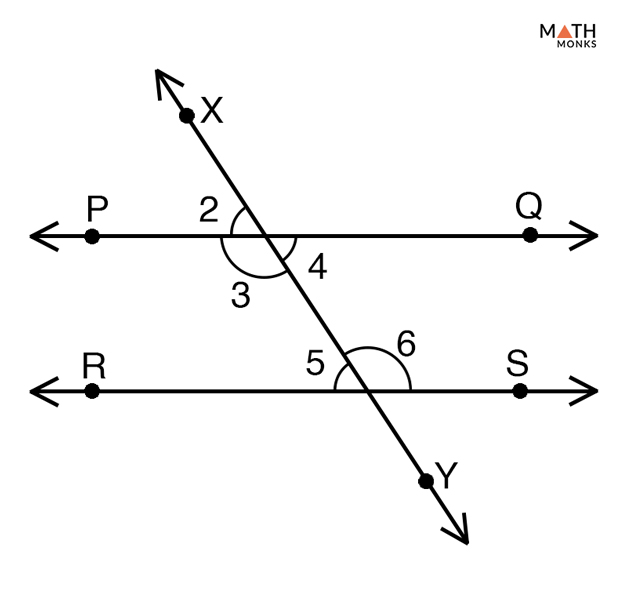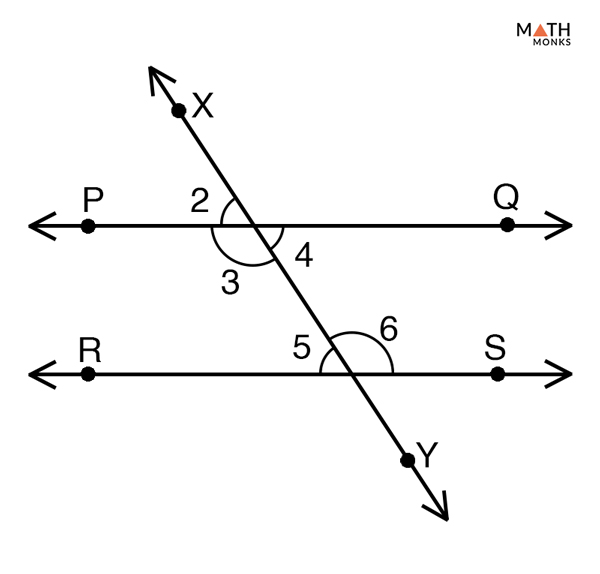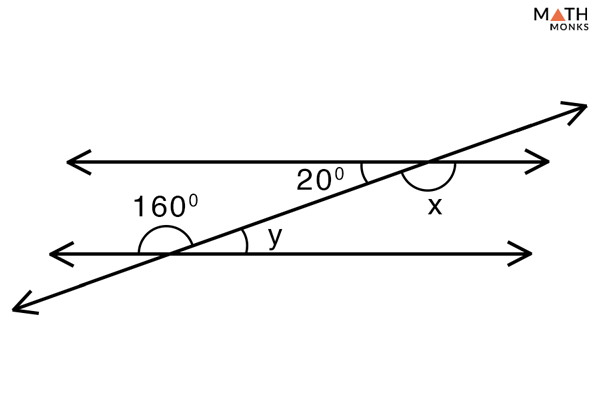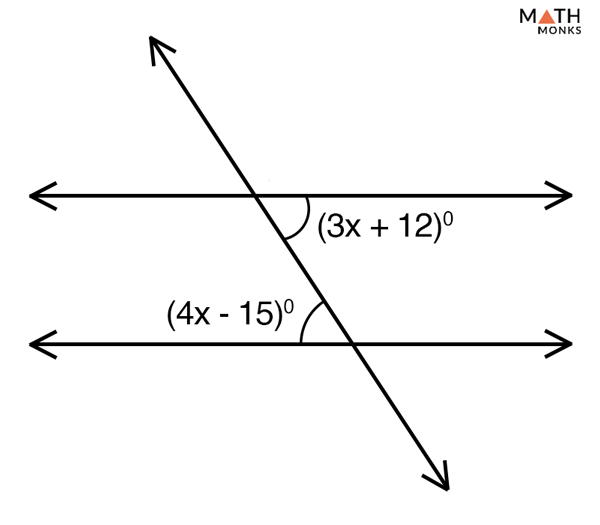# Alternate Interior Angles

## What are Alternate Interior Angles

Alternate interior angles are the pairs of angles formed when a transversal intersects two parallel or non-parallel lines. They are formed on the inner side of the parallel lines but on the opposite sides of the transversal.

If the alternate interior angles are equal the two lines intersected by the transversal are parallel to each other. On the other hand, alternate interior angles formed when a transversal crosses two non-parallel lines, are found to have no geometric relation.

The above figure shows two parallel lines AB and CD intersected by the transversal RS. The two pairs of alternate interior angles formed are:

• ∠1 and 2
• ∠3 and ∠4

Thus ∠1 = ∠2 and ∠3 = ∠4

## Properties

1. They are congruent
2. Angles formed on the same side of the transversal involving two parallel lines are supplementary. These are known as consecutive interior angles
3. In the case of non-parallel lines, alternate interior angles have no geometric relation with each other
4. They are supplementary if the transversal intersects two parallel lines at right angles

## Alternate Interior Angles TheoremProve Alternate Interior Angles Theorem

To prove:

∠3 = ∠6 and ∠4 = ∠5

Proof:

Let ‘PQ’ and ‘RS’ be the two parallel lines intersected by the transversal XY
From the properties of parallel lines, we know that if a transversal intersects two parallel lines then the corresponding angles and the vertically opposite ages are equal to each other.
Thus, we can write
∠2 = ∠5…… (1) (Corresponding angles)
∠2 = ∠4…… (2) (Vertically opposite angles)
From (1) and (2) we get,
∠4 = ∠5 (Alternate interior angles)
Similarly,
∠3 = ∠6
Hence Proved

### The converse of Alternate Interior Angles TheoremProve the Converse of Alternate Interior Angles Theorem

To prove:

PQ∥ RS

Proof:

Let ∠4 = ∠5 and ∠3 = ∠6 be the two pairs of congruent alternate interior angles
Using the above figure, we can write
∠2 = ∠5 (Corresponding angles)
Hence PQ is parallel to RS
Hence Proved

Let us take some examples to understand the concept better.Find the value of x and y in the given figure.

Solution:

As, ∠20° = ∠y (Alternate interior angles)
Hence, ∠y =20°
Similarly,
∠160° = ∠x (Alternate interior angles)
Hence, ∠x =160°Solve the value of x in the given figure.

Solution:

As we know the alternate interior angles are congruent
Thus,
(4x – 15)° = (3x + 12)°
4x – 3x = 12 + 15
x = 27

Given that the two alternate interior angles (4x – 19)° and (3x + 16)° are congruent. Find the value of x and the values of the two alternate interior angles.

Solution:

According to the interior angle theorem, alternate interior angles are equal when the transversal crosses two parallel lines.
Thus,
(4x – 19)° = (3x + 16)°
4x – 3x = 16 + 19
x = 35°
Now, substituting the value of x in both the interior angles expression we get,
(4x – 19)° = 4 x 35 – 19 = 121°
(3x + 16)° = 3 x 35 + 16 = 121°
Thus the values of the two alternate interior angles are 121°

## Examples in Real Life

• The letter ‘Z’ where the top and the bottom horizontal lines are parallel and the diagonal line is the transversal
• Windows with panes divided by muntins